## Explanation of Valence Bond Theory

Valence bond theory states that overlap between two atomic orbitals forms a covalent bond between two atoms.

### Learning Objectives

Describe valence bond theory

### Key Takeaways

#### Key Points

• Valence bond and molecular orbital theories are used to explain chemical bonding.
• Two atoms that have unpaired electrons in their orbitals can overlap to give rise to a chemical bond.
• A sigma bond ($\sigma$) forms when two atomic orbitals overlap between the nuclei of two atoms (also known as the internuclear axis ).
• Pi bonds ($\pi$) occur when two atomic orbitals overlap outside of the space between the nuclei (outside of the internuclear axis).
• The strongest bonds form with maximum overlap between orbitals.

#### Key Terms

• covalent bond: a chemical bond that is formed from the sharing of two electrons between two atoms.
• internuclear axis: The space between the nuclei of two atoms bonded to each other.
• atomic orbitals: the physical region or space where an electron may be present or found, with a probability calculated from the precise mathematical form of the orbital.
• valence bond theory: a covalent bond forms between the two atoms by the overlap of half-filled valence atomic orbitals from each atom.

In chemistry, valence bond (VB) theory is one of two basic theories—along with molecular orbital (MO) theory—that use quantum mechanics to explain chemical bonding. According to VB theory, a covalent bond forms from the physical overlap of half-filled valence orbitals in two atoms.

### Mechanism of Bonding in VB Theory

The VB theory describes the formation of covalent bonds from the overlap of atomic orbitals on two different atoms. Because of the overlap, it is highly probable that a pair of electrons are found in the physical region or space where the orbitals overlap.

### Sigma ($\sigma$) and Pi ($\pi$) Bonds

There are two types of overlapping orbitals: sigma ($\sigma$) and pi ($\pi$). Both bonds are formed from the overlap of two orbitals, one on each atom. $\sigma$ bonds occur when orbitals overlap between the nuclei of two atoms, also known as the internuclear axis.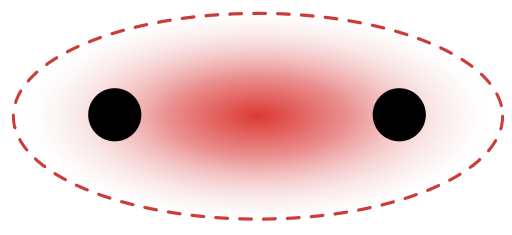$\sigma$ Bond Formation: Atomic orbitals from two atoms overlap in the region between the nuclei (internuclear axis). Therefore, the resulting electron density of the shared electrons lies in the red region shown in the image.

$\pi$ bonds occur when two (unhybridized) p-orbitals overlap. The p-orbitals, in one $\pi$ bond, are located above and below the nuclei of the atoms. By occupying the region of space that is above, below, and on the sides of an atom’s nuclei, two $\pi$ bonds can form.$\pi$ Bond Formation: Two unhybridized p-orbitals can overlap so that the electron density of the shared electron pair is described by the $\pi$ bond depicted on the right.

Both types of overlapping orbitals can be related to bond order. Single bonds have one sigma bond. Double bonds consist of one $\sigma$ and one $\pi$ bond, while triple bonds contain one $\sigma$ and two $\pi$ bonds.

### Comparing VB and MO

VB theory complements molecular orbital (MO) theory, which does not adhere to the VB concept that electron pairs are localized between two specific atoms in a molecule. MO theory states that electrons are distributed in sets of molecular orbitals that can extend over the entire molecule. MO theory can predict magnetic and ionization properties in a straightforward manner. VB theory produces similar results, but is more complicated.

### Bond Character

An important aspect of the VB theory is the condition of maximum overlap which leads to the formation of the strongest possible bonds. This theory is used to explain the covalent bond formation in many molecules. In the F2 molecule, the F–F $\sigma$ covalent bond is formed by the overlap of pz orbitals of the two F atoms, each containing an unpaired electron. Since the nature of the overlapping orbitals is different in H2 and F2 molecules, bond strength and bond lengths differ between H2 and F2 molecules.

In an HF molecule, the covalent $\sigma$ bond forms from the overlap of the 1s orbital of H and the 2pz orbital of F, each containing an unpaired electron. Mutual sharing of electrons between H and F results in a covalent bond in HF.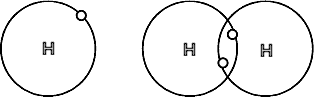Covalent bond between hydrogen atoms: Each hydrogen atom has one electron. To complete their valence shells, they bond and share one electron with each other. This allows electrons to move about both atoms and gives both atoms access to two electrons; they become a stable H2 molecule joined by a single covalent bond.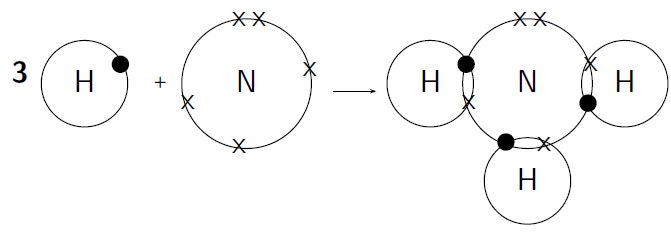Covalent bonding in a molecule of ammonia: Each hydrogen atom needs one more electron to complete its valence energy shell. The nitrogen atom needs three more electrons to complete its valence energy shell. Therefore, three pairs of electrons must be shared between the four atoms involved. The nitrogen atom will share three of its electrons so that each of the hydrogen atoms now has a complete valence shell. Each of the hydrogen atoms will share its electron with the nitrogen atom to complete its valence shell.

## sp3 Hybridization

sp3 hybrid orbitals form when a single s and three p orbitals hybridize.

### Learning Objectives

Explain the process of hybridization as it applies to the formation of sp3 hybridized atoms.

### Key Takeaways

#### Key Points

• The bonds in a methane (CH4) molecule are formed by four separate but equivalent orbitals; a single 2s and three 2p orbitals of the carbon hybridize into four sp3 orbitals.
• In the ammonia molecule (NH3), 2s and 2p orbitals create four sp3 hybrid orbitals, one of which is occupied by a lone pair of electrons.
• In a water molecule, two sp3 hybrid orbitals are occupied by the two lone pairs on the oxygen atom, while the other two bond with hydrogen.

#### Key Terms

• tetravalent: having a valence of 4

In a tetravalent molecule, four outer atoms are bonded to a central atom. Perhaps the most common and important example of this bond type is methane, CH4. In the ground state of the free carbon atom, there are two unpaired electrons in separate 2p orbitals. To form four bonds, the atom must have four unpaired electrons; this requires that carbon’s valence 2s and 2p orbitals each contain an electron for bonding.

The single 2s orbital is spherical, different from the dumbbell-shaped 2p orbitals. This would indicate that one of the four bonds differs from the other three, but scientific tests have proven that all four bonds have equal length and energy; this is due to the hybridization of carbon’s 2s and 2p valence orbitals.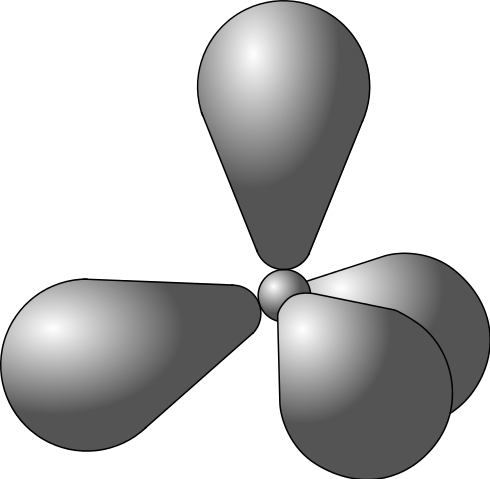Methane: The methane molecule has four equal bonds.

In hybridization, carbon’s 2s and three 2p orbitals combine into four identical orbitals, now called sp3 hybrids.

The bonds between carbon and hydrogen can form the backbone of very complicated and extensive chain hydrocarbon molecules. The simplest of these is ethane (C2H6), in which an sp3 orbital on each of the two carbon atoms joins (overlaps) to form a carbon-carbon bond; then, the remaining carbon sp3 orbital overlaps with six hydrogen 1s orbitals to form the ethane molecule.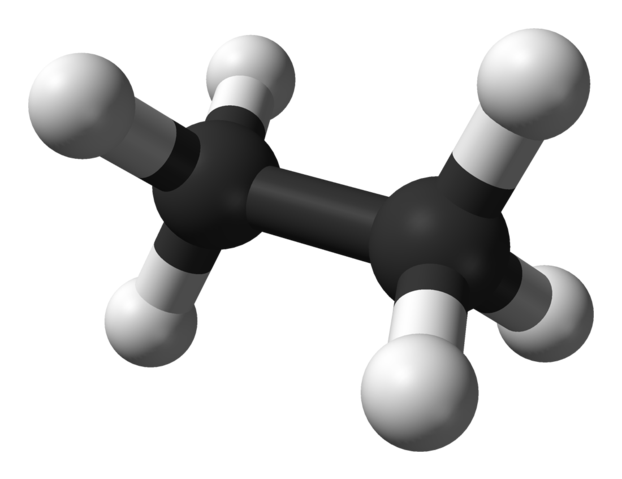Ethane: Ethane can form by replacing one of the hydrogen atoms in CH4 with another sp3 hybridized carbon fragment.

If lone electron pairs are present on the central atom, thet can occupy one or more of the sp3 orbitals. For example, in the ammonia molecule, the fourth of the sp3 hybrid orbitals on the nitrogen contains the two remaining outer-shell electrons, which form a non-bonding lone pair.

In the water molecule, the oxygen atom can form four sp3 orbitals. Two of these are occupied by the two lone pairs on the oxygen atom, while the other two are used for bonding. The observed H-O-H bond angle in water (104.5°) is less than the tetrahedral angle (109.5°); one explanation for this is that the non-bonding electrons tend to remain closer to the central atom and thus exert greater repulsion on the other orbitals, pushing the two bonding orbitals closer together.

## sp2 Hybridization

sp2 hybridization occurs between one s-orbital and two p-orbitals.

### Learning Objectives

Recognize the role of sp2 hybridized atoms in sigma and pi bonding.

### Key Takeaways

#### Key Points

• For boron to bond with three fluoride atoms in boron trifluoride (BF3), the atomic s- and p- orbitals in boron’s outer shell mix to form three equivalent sp2 hybrid orbitals.
• In an ethene molecule, a double bond between carbons forms with one sigma and one pi bond.
• The sigma bond in the C=C for ethene forms between two sp2 hybrid orbitals of two carbon atoms, and a pi bond for between two p orbitals.

#### Key Terms

• sp2 hybridization: the 2s orbital mixes with only two of the three available 2p orbitals
• hybridization: mixing atomic orbitals into new hybrid orbitals, suitable for pairing electrons to form chemical bonds in valence bond theory

Boron trifluoride (BF3) has a boron atom with three outer-shell electrons in its normal or ground state, as well as three fluorine atoms, each with seven outer electrons. One of the three boron electrons is unpaired in the ground state. In order to explain the bonding, the 2s orbital and two of the 2p orbitals (called sp2 hybrids) hybridize; one empty p-orbital remains.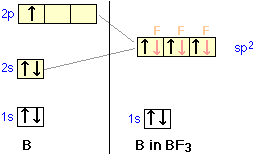Boron configuration diagram: One of the three boron electrons is unpaired in its ground state. The atomic s- and p-orbitals in boron’s outer shell mix to form three equivalent hybrid orbitals. These particular orbitals are called sp2 hybrids, meaning that this set of orbitals derives from one s- orbital and two p-orbitals of the free atom.The Lewis structure for ethene: The carbon atoms are sp2 hybridized. Two sp2 hybrids bond with the hydrogen atoms, and the other forms a sigma bond with the other carbon atom. The p-orbitals that are unused by the carbon atoms in the hybridization overlap to form the C=C.

### sp2 Hybridization in Ethene and the Formation of a Double Bond

Ethene (C2H4) has a double bond between the carbons. In this case, carbon will sp2 hybridize; in sp2 hybridization, the 2s orbital mixes with only two of the three available 2p orbitals, forming a total of three sp hybrid orbitals with one p-orbital remaining. The three hybridized orbitals explain the three sigma bonds that each carbon forms.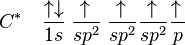sp2 hybridization in ethene: In sp^2 hybridization, the 2s orbital mixes with only two of the three available 2p orbitals, forming a total of three sp^2 orbitals with one p-orbital remaining.

The two carbon atoms form a sigma bond in the molecule by overlapping two sp2 orbitals. Each carbon atom forms two covalent bonds with hydrogen by s–sp2 overlap, all with 120° angles. The pi bond between the carbon atoms perpendicular to the molecular plane is formed by 2p–2p overlap.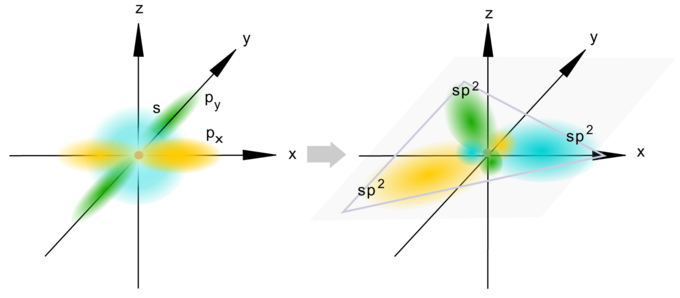Formation of sp2 hybrid orbitals: This illustration shows how an s-orbital mixes with two p orbitals to form a set of three sp2 hybrid orbitals. Notice again how the three atomic orbitals yield the same number of hybrid orbitals.

## sp Hybridization

sp hybrid orbitals form from one s-orbital and one p-orbital.

### Learning Objectives

Describe the bonding geometry of an sp hybridized atom.

### Key Takeaways

#### Key Points

• In sp  hybridization, the s orbital overlaps with only one p orbital.
• Any central atom surrounded by just two regions of valence electron density in a molecule will exhibit sp hybridization.
• sp orbitals are oriented at 180 degrees to each other.

#### Key Terms

• hybrid orbital: formed by combining multiple atomic orbitals on the same atom
• sp hybrid: an orbital formed between one s-orbital and one p-orbital

In sp hybridization, the s orbital overlaps with only one p orbital. Atoms that exhibit sp hybridization have sp orbitals that are linearly oriented; two sp orbitals will be at 180 degrees to each other.

Any central atom surrounded by just two regions of valence electron density in a molecule will exhibit sp hybridization. Some examples include the mercury atom in the linear HgCl2 molecule, the zinc atom in Zn(CH3)2, which contains a linear C–Zn–C arrangement, the carbon atoms in HCCH and CO2, and the Be atom in BeCl2. This last example will be discussed in more detail below.

### sp Hybridization in BeCl2

The beryllium atom in a gaseous BeCl2 molecule is an example of a central atom with no lone pairs of electrons in a linear arrangement of three atoms. There are two regions of valence electron density in the BeCl2 molecule that correspond to the two covalent Be–Cl bonds. To accommodate these two electron domains, two of the Be atom’s four valence orbitals will mix to yield two hybrid orbitals.

When atomic orbitals hybridize, the valence electrons occupy the newly created orbitals. The Be atom had two valence electrons, so each of the sp orbitals gets one of these electrons. Each of these electrons pairs up with the unpaired electron on a chlorine atom when a hybrid orbital and a chlorine orbital overlap during the formation of the Be–Cl bonds.

The hybridization process involves mixing of the valence s orbital with one of the valence p orbitals to yield two equivalent sp hybrid orbitals that are oriented in a linear geometry.s and p Orbitals Combining to Form Two sp Orbitals: Hybridization of an s orbital and a p orbital of the same atom produces two sp hybrid orbitals. Each hybrid orbital is oriented primarily in just one direction. Note that each sp orbital contains one lobe that is significantly larger than the other. The set of two sp orbitals are oriented at 180°, which is consistent with the geometry for two domains.

The set of sp orbitals appears similar in shape to the original p orbital, but there is an important difference. The number of atomic orbitals combined always equals the number of hybrid orbitals formed. The p orbital is one orbital that can hold up to two electrons. The sp set is two equivalent orbitals that point 180° from each other. The two electrons that were originally in the s orbital are now distributed to the two sp orbitals, which are half filled.

In gaseous BeCl2, these half-filled hybrid orbitals will overlap with orbitals from the chlorine atoms to form two identical σ bonds.

### Energy Level Diagrams for sp Orbitals

The electronic differences in an isolated Be atom and in the bonded Be atom can be illustrated using an orbital energy-level diagram. These diagrams represent each orbital by a horizontal line (indicating its energy) and each electron by an arrow. Energy increases toward the top of the diagram. We use one upward arrow to indicate one electron in an orbital and two arrows (up and down) to indicate two electrons of opposite spin.Orbital Energy-Level Diagrams For Isolated and Bonded Be: The left orbital energy-level diagram shows both electrons of Be in the 2s orbital. The right energy-level diagrams shows sp hybridized orbitals on Be in the linear BeCl2 molecule. Each of the two sp hybrid orbitals holds one electron and is thus half filled and available for bonding via overlap with a Cl 3p orbital.

## Hybridization in Molecules Containing Double and Triple Bonds

sp2, sp hybridizations, and pi-bonding can be used to describe the chemical bonding in molecules with double and triple bonds.

### Learning Objectives

Describe the role of hybridization in the formation of double and triple bonds.

### Key Takeaways

#### Key Points

• A new hybrid orbital forms when atomic orbitals are mixed; this process is called hybridization.
• The bonding in ethene (which contains a C=C) occurs due to sp2 hybridization in each of the carbon atoms.
• Molecules with triple bonds, such as acetylene, have two pi bonds and one sigma bond.

#### Key Terms

• hybridisation: mixing atomic orbitals to form new hybrid orbitals suitable for the qualitative description of atomic bonding properties
• triple bond: a covalent bond in which three electron pairs (instead of the usual one) are shared between two atoms; most common between carbon atoms and carbon or nitrogen atoms; symbolized in formulae as ≡
• pi bond: covalent chemical interactions where two lobes of one involved atomic (p) orbital overlap two lobes of the other involved atomic (p) orbital
• double bond: a covalent bond in which two electron pairs (instead of the usual one) are shared between two atoms; most common between carbon atoms and carbon, oxygen, or nitrogen atoms

In chemistry, hybridization is the concept of mixing atomic orbitals to form new hybrid orbitals suitable for describing bonding properties. Hybridized orbitals are very useful in explaining of the shape of molecular orbitals for molecules, and are an integral part of valence bond theory.

The hybrids are named for the atomic orbitals involved in the hybridization. In methane (CH4) for example, a set of sp3 orbitals forms by mixing one s- and three p-orbitals on the carbon atom. The orbitals are directed toward the four hydrogen atoms, which are located at the vertices of a regular tetrahedron.

Ethene (C2H4) has a double bond between the carbons. For this molecule, carbon will sp2 hybridize. In sp2 hybridization, the 2s orbital mixes with only two of the three available 2p orbitals, forming a total of 3 sp2 orbitals with one p-orbital remaining. In ethylene (ethene), the two carbon atoms form a sigma bond by overlapping two sp2 orbitals; each carbon atom forms two covalent bonds with hydrogen by s–sp2 overlapping all with 120° angles. The pi bond between the carbon atoms forms by a 2p-2p overlap. The hydrogen-carbon bonds are all of equal strength and length, which agrees with experimental data.

Multiple bonds can also occur between dissimilar atoms. When the two O-atoms are brought up to opposite sides of the carbon atom in carbon dioxide, one of the p orbitals on each oxygen forms a pi bond with one of the carbon p-orbitals. In this case, sp hybridization leads to two double bonds.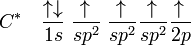sp2 hybridization: In ethene, carbon sp2 hybridizes, because one π (pi) bond is required for the double bond between the carbons, and only three σ bonds form per carbon atom.Ethene structure: Ethene has a double bond between the carbons.

sp hybridization explains the chemical bonding in compounds with triple bonds, such as alkynes; in this model, the 2s orbital mixes with only one of the three p-orbitals, resulting in two sp orbitals and two remaining p-orbitals. The chemical bonding in acetylene (ethyne) (C2H2) consists of sp-sp overlap between the two carbon atoms forming a sigma bond, as well as two additional pi bonds formed by p-p overlap. Each carbon also bonds to hydrogen in a sigma s-sp overlap at 180° angles.Lewis structure of ethyne, which contains a triple bond: The sp hybridized orbitals are used to overlap with the 1s hydrogen orbitals and the other carbon atom. The remaining, non-hybridized p-orbitals overlap for the double and triple pi bonds.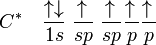sp hybridisation: In this model, the 2s orbital mixes with only one of the three p-orbitals, resulting in two sp-orbitals and two remaining unchanged p-orbitals.